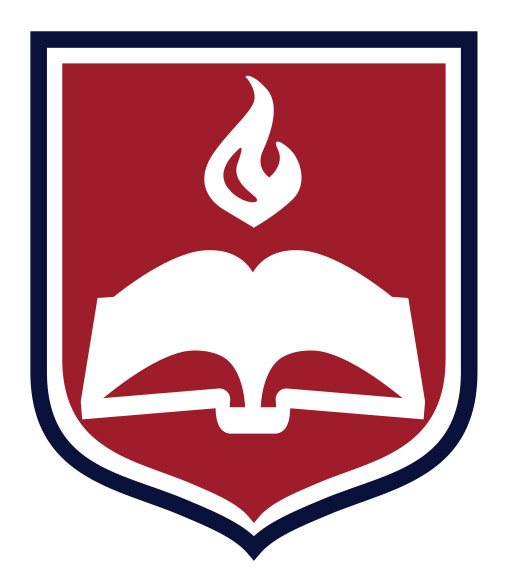## Mathematics

### Course Descriptions

MA101 College Math Survey – This study will include functions and algebraic expressions, graphs, quadratic equations, exponents and radicals, and inequalities.
One semester, three hours

MA201 Advanced Math I – This course provides in-depth coverage of trigonometry, logarithms, analytic geometry, upper-level algebraic concepts, and practice of intermediate algebraic concepts and trigonometry.
One semester, three hours Prerequisite – MA101

MA202 Advanced Math II – This course includes lessons on functions, matrices, statistics, and use of the graphing calculator as well as pre-calculus concepts.
One semester, three hours Prerequisite – MA201

MA220 Trigonometry – This course is a study of trigonometric functions, identities, graphs, solutions to triangles, exponential and logarithmic functions, and some advanced college algebra.
One semester, three hours Prerequisite – MA101

MA230 College Geometry – This course is an introduction to advanced Euclidean geometry with topics including circles, constructions, loci, polygons, triangles, and congruence.
One semester, three hours Prerequisite – MA101

MA300 Pre-Calculus – This course is a review of algebra, geometry, trigonometry concepts with a heavy emphasis placed on common and natural logarithms, trigonometric and exponential functions, logarithmic equations, factorials, and binomial theorems and limits.
One semester, three hours Prerequisites – MA202, MA220, MA230

MA301 Calculus – This course is a study of functions, limits, derivatives of algebraic functions, and definite and indefinite integrals with their many applications.
One semester, three hours Prerequisite – MA300

MA302 Analytic Geometry – This course studies the transcendental and hyperbolic functions, methods of integration, and introduction to vectors.
One semester, three hours Prerequisites – MA230, MA301

MA403 Abstract Algebra – This course is a study including set theory, groups, rings, fields, ideals, unique factorization domains, and other topics. Ideas are illustrated with real numbers, modular arithmetic, and other representations.
One semester, three hours Prerequisite – MA202